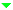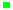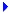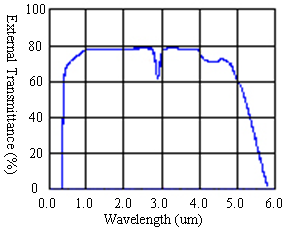Optical MaterialsOptical GlassLow Expansion MaterialsIR MaterialsCrystalsMicro-Optical ComponentsFiber Optic ComponentsLight Sources & PhotodiodesSpectrometersWafersServices

Lithium Niobate (LiNbO3)

Lithium Niobate, a well-developed optical material, has been widely used for surfaced acoustic devices, integrated optic devices, and new wavelength generation and refractive elements. The wide applications are due to its fundamental properties including high electro-optic and nonlinear optic coefficients, a wide transparency range, high electro-mechanical coupling coefficients, and excellent chemical and mechanical stability.Figure 1: External transmittance curve for lithium niobate crystal

 Crystal Structure Trigonal lattice constant (Å) a = 5.148, c = 13.863 Melting Point (°C) 1255 ± 5 Curie Point (°C) 1140 ± 5 Mohs Hardness 5 Density (g/cm^3) 4.64 Absorption Coefficient (/cm) α<0.001  at 1064nm Hygroscopic Susceptibility No Relative Dielectric Constant εT11 /εo= 85, εT33 /εo= 29.5 Linear Coefficient of Thermal Expansion (25°C)  ( /°C) ||C: 7.5X10-6⊥C: 15X10-6 Optical Homogeneity (/cm) ≈ 5×10-5 Transmission Range (nm) 420 ~ 5200 Damage Threshold (MW/cm2) 100  at 1064nm (10ns) Refractive Indices no = 2.220, ne = 2.146 at 1300 nm no = 2.232, ne = 2.156 at 1064nm no = 2.268, ne = 2.203 at 632.8nm Sellmeier Equations (λ in um) no2 = 4.9048+0.11768/(λ2-0.04750)-0.027169×λ2 ne2 = 4.5820+0.099169/(λ2-0.04443)-0.021950×λ2 Nonlinear Optical Coefficients (pm/V) d33=34.4, d31=d15=5.95, d22=3.07 Electro-optic Coefficients (pm/V at 633nm) r33 = 32, r13 = 10, r22 = 6.8, r51=32 (low frequency) r33 = 31, r13 = 8.6, r22 = 3.4, r51=28 (high frequency) Table 1: LiNbO3 Properties